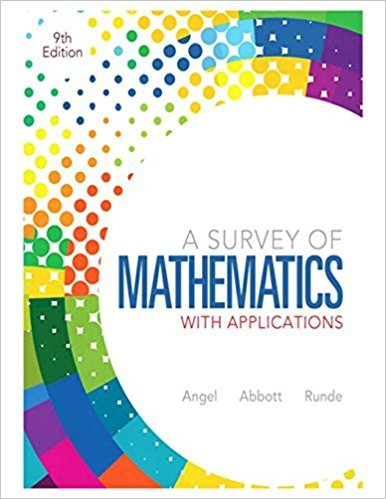×
×

# Solutions for Chapter 14.2: Graph Theory## Full solutions for A Survey of Mathematics with Applications | 9th Edition

ISBN: 9780321759665Solutions for Chapter 14.2: Graph Theory

Solutions for Chapter 14.2
4 5 0 401 Reviews
13
0
##### ISBN: 9780321759665

A Survey of Mathematics with Applications was written by and is associated to the ISBN: 9780321759665. This textbook survival guide was created for the textbook: A Survey of Mathematics with Applications, edition: 9. Chapter 14.2: Graph Theory includes 58 full step-by-step solutions. This expansive textbook survival guide covers the following chapters and their solutions. Since 58 problems in chapter 14.2: Graph Theory have been answered, more than 75043 students have viewed full step-by-step solutions from this chapter.

Key Math Terms and definitions covered in this textbook
• Column picture of Ax = b.

The vector b becomes a combination of the columns of A. The system is solvable only when b is in the column space C (A).

• Complete solution x = x p + Xn to Ax = b.

(Particular x p) + (x n in nullspace).

• Distributive Law

A(B + C) = AB + AC. Add then multiply, or mUltiply then add.

• Ellipse (or ellipsoid) x T Ax = 1.

A must be positive definite; the axes of the ellipse are eigenvectors of A, with lengths 1/.JI. (For IIx II = 1 the vectors y = Ax lie on the ellipse IIA-1 yll2 = Y T(AAT)-1 Y = 1 displayed by eigshow; axis lengths ad

• Exponential eAt = I + At + (At)2 12! + ...

has derivative AeAt; eAt u(O) solves u' = Au.

• Four Fundamental Subspaces C (A), N (A), C (AT), N (AT).

Use AT for complex A.

• Fourier matrix F.

Entries Fjk = e21Cijk/n give orthogonal columns FT F = nI. Then y = Fe is the (inverse) Discrete Fourier Transform Y j = L cke21Cijk/n.

• Full column rank r = n.

Independent columns, N(A) = {O}, no free variables.

• Fundamental Theorem.

The nullspace N (A) and row space C (AT) are orthogonal complements in Rn(perpendicular from Ax = 0 with dimensions rand n - r). Applied to AT, the column space C(A) is the orthogonal complement of N(AT) in Rm.

• Hankel matrix H.

Constant along each antidiagonal; hij depends on i + j.

• Hermitian matrix A H = AT = A.

Complex analog a j i = aU of a symmetric matrix.

• Kronecker product (tensor product) A ® B.

Blocks aij B, eigenvalues Ap(A)Aq(B).

• Linear combination cv + d w or L C jV j.

• Multiplication Ax

= Xl (column 1) + ... + xn(column n) = combination of columns.

• Nilpotent matrix N.

Some power of N is the zero matrix, N k = o. The only eigenvalue is A = 0 (repeated n times). Examples: triangular matrices with zero diagonal.

• Nullspace N (A)

= All solutions to Ax = O. Dimension n - r = (# columns) - rank.

• Saddle point of I(x}, ... ,xn ).

A point where the first derivatives of I are zero and the second derivative matrix (a2 II aXi ax j = Hessian matrix) is indefinite.

• Similar matrices A and B.

Every B = M-I AM has the same eigenvalues as A.

• Spectrum of A = the set of eigenvalues {A I, ... , An}.

Spectral radius = max of IAi I.

• Stiffness matrix

If x gives the movements of the nodes, K x gives the internal forces. K = ATe A where C has spring constants from Hooke's Law and Ax = stretching.

×